# Turn a string into a formula with EvaluateI encountered a situation recently where I needed to convert a text string into a formula for calculation.  I was expecting to find Excel would have the ability to do this.  And it does . . . in part.

If you have a number stored as text you can multiply it by 1 and it will be converted to a number.  But what if we want to convert a whole text string into a formula, rather than just converting a single number?  How can we achieve this?  Excel has the EVALUATE formula, however, this is a legacy formula which does not quite function how you might Expect.  So, let’s see how we can solve this.

## Using EVALUATE to convert string to a formula

Let’s use an easy example of how the EVALUATE function might convert a string into a formula.

In our worksheet we have data in 3 cells

A1 = 1
A2 = +
A3 = 1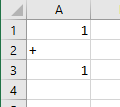If we wanted to use this information to create the formula 1+1 (which equals 2), how could we do it?  The EVALUATE formula would be the perfect option, except it only partially exists.  If we were to include a formula such as:

=EVALUATE(A1&A2&A3)Note: The & is used to combine cells together into a single string, so our example would become:

=EVALUATE(“1+1”)

It seems like it might work, but if we try, we will receive an error like this: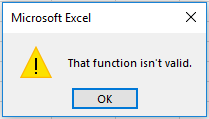However, we can create that same formula as a named range.

Formulas -> Define Names -> Name Manager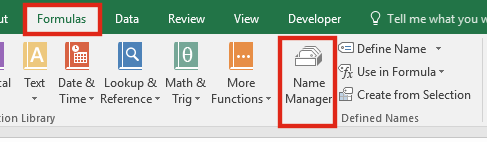Click NewEnter the formula in the Refers To box and click OK.That named range is now available for use in formulas.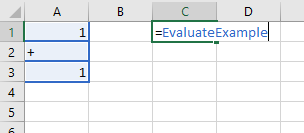The result in Cell C1 will become: 2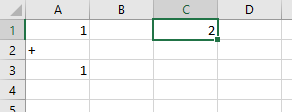This might be useful to a certain extent, but in most circumstances it does not provide a suitable solution for converting a text string to a formula.  We need something which gives the same result, but which can be used as a normal Excel formula.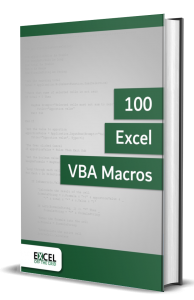• Contains 100 Excel VBA macros
• Learn VBA by following along with the example codes
• Apply to your macros, automate Excel, save time.## EVALUATE as a User Defined Function to convert string to formula

It is possible to create our own Excel functions with VBA; these are known as a User Defined Functions (UDF).  These work the same way as Excel’s standard functions, but we determine how the result should be calculated.

If we insert the following VBA code into a Module of the Visual Basic Editor we can create our own EVALUATE formula.

```Function EvaluateString(strTextString As String)
Application.Volatile
EvaluateString = Evaluate(strTextString)
End Function```

That’s it.  Just 4 lines.  Even though Excel does not have the EVALUATE function itself, VBA still does.

We are now able to use this as if it were a normal function.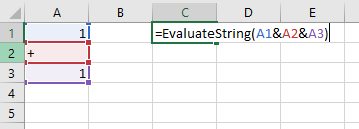The result in Cell C1 will become: 2There is no need to create a named range each time, and this formula is now available for use in our workbook.

Note: If the code is not placed in a Module it will not work.

## Conclusion

To convert a text string to a formula it appears the VBA User Defined Function route is the best option.  Whilst it is possible to use a named range, it is restricted by what it can achieve.

Don’t forget:

I’m guessing the examples in this post didn’t exactly meet your situation.  We all use Excel differently, so it’s impossible to write a post that will meet everybody’s needs.  By taking the time to understand the techniques and principles in this post (and elsewhere on this site) you should be able to adapt it to your needs.

But, if you’re still struggling you should:

1. Read other blogs, or watch YouTube videos on the same topic.  You will benefit much more by discovering your own solutions.
2. Ask the ‘Excel Ninja’ in your office.  It’s amazing what things other people know.
3. Ask a question in a forum like Mr Excel, or the Microsoft Answers Community.  Remember, the people on these forums are generally giving their time for free.  So take care to craft your question, make sure it’s clear and concise.  List all the things you’ve tried, and provide screenshots, code segments and example workbooks.
4. Use Excel Rescue, who are my consultancy partner.   They help by providing solutions to smaller Excel problems.

What next?
Don’t go yet, there is plenty more to learn on Excel Off The Grid.  Check out the latest posts:

Save

## 6 thoughts on “Turn a string into a formula with Evaluate”

1.Gary says:

Thanks, that is a life saving tip

2.Excel Off The Grid says:

Thanks Gary.

I still find it odd that there is not a native function in Excel which does this. If there is we’ve obviously both missed it. 🙂

3.Nat says:

Mine just returns 0, not even the example works, zero is all I get with the 🙁

•Excel Off The Grid says:

Hi Nat – I’ve re-tried the examples, and they still work. It might be worth checking that you’ve applied it correctly.

•Steve81uk says:

I know this may be a little late, but this only thing that I would suggest is that it has cannot be done as a range and each cell selected separately i.e A1, A2, A3, and not A1:A3 .. That may help.

Awesome work by the way. it works for me on the simpler codes. but am trying to force through a calculated string as a formula. I have managed to make a calculated variable formula that is a sumproduct, so that I can get the values from various files. almost there just needing to get my string of =sumproduct(…..) back as a normal formula output. Maybe it’s just impossible. but I feel its worth a shot. (doing this as a workaround of =sumproduct(Indirect(…)) as this doesn’t work.

4.Gian Maria says:

OK, but if I build a formula into a SQL Query and a field of resultset contains a string that is a formula. How do I get it recognized by Excel?
Thanks